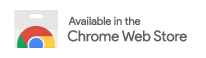# Pre-university Mathematics

A selection of recordings suitable for students with some mathematical background, such as A-level maths students.

Education 4 résziPod version - Beyond Infinity? Dr Joel Feinstein
24 perc 2. rész
This popular maths talk by Dr Joel Feinstein gives an introduction to various different kinds of infinity, both countable and uncountable. These concepts are illustrated in a somewhat informal way using the notion of Hilbert's infinite hotel. In this talk, the hotel manager tries to fit various infinite collections of guests into the hotel. The students should learn that many apparently different types of infinity are really the same size. However, there are genuinely "more" real numbers than theiPod version - Why do we do proofs? - Dr Joel Feinstein
30 perc 1. rész
This is the first of three sessions by Dr Joel Feinstein on how and why we do proofs. Dr Feinstein's blog is available at http://explainingmaths.wordpress.com/ The aim of this session is to motivate students to understand why we might want to do proofs, why proofs are important, and how they can help us. In particular, the student will learn the following: proofs can help you to really see WHY a result is true; problems that are easy to state can be hard to solve (Fermat's Last Theorem); soBeyond Infinity? Dr Joel Feinstein
24 perc 4. rész
This popular maths talk by Dr Joel Feinstein gives an introduction to various different kinds of infinity, both countable and uncountable. These concepts are illustrated in a somewhat informal way using the notion of Hilbert's infinite hotel. In this talk, the hotel manager tries to fit various infinite collections of guests into the hotel. The students should learn that many apparently different types of infinity are really the same size. However, there are genuinely "more" real numbers than theWhy do we do proofs? - Dr Joel Feinstein
30 perc 3. rész
This is the first of three sessions by Dr Joel Feinstein on how and why we do proofs. Dr Feinstein's blog is available at http://explainingmaths.wordpress.com/ The aim of this session is to motivate students to understand why we might want to do proofs, why proofs are important, and how they can help us. In particular, the student will learn the following: proofs can help you to really see WHY a result is true; problems that are easy to state can be hard to solve (Fermat's Last Theorem); soSpeed: